# Basic Electronics – Hall Effect

Hall Effect was named after Edwin Hall, its discoverer. This is somewhat similar to Fleming`s right hand rule. When a current carrying conductor I is placed in a transverse magnetic field B, an electric field E is induced in the conductor perpendicular to both I and B. This phenomenon is called as Hall Effect.

### Explanation

When a current carrying conductor is placed in a transverse magnetic field, then this magnetic field exerts some pressure on the electrons which take a curved path to continue their journey. The conductor with energy applied is shown in the following figure. The magnetic field is also indicated.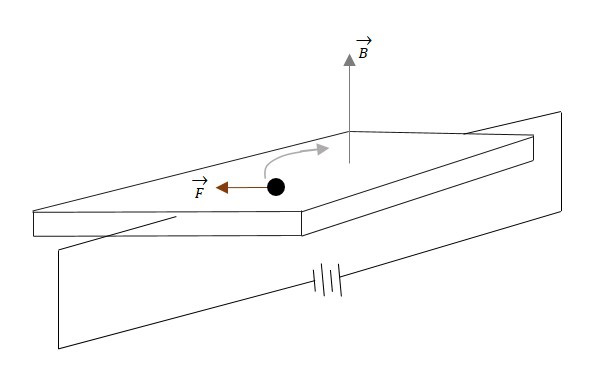As electrons travel through the conductor that lies in a magnetic field B, the electrons will experience a magnetic force. This magnetic force will cause the electrons to travel close to one side than the other. This creates a negative charge on one side and positive charge on the other, as shown in the following figure.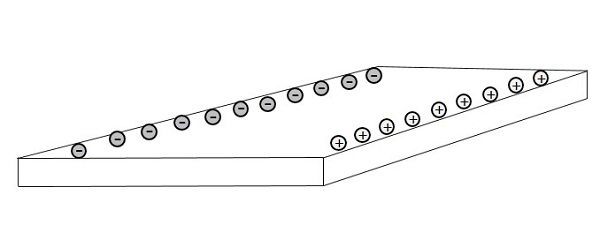This separation of charge will create a voltage difference which is known as Hall Voltage or Hall EMF. The voltage builds up until the electric field produces an electric force on the charge that is equal and opposite of the magnetic force. This effect is known as Hall Effect.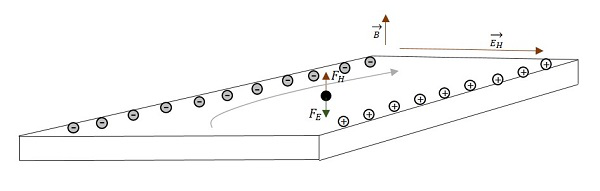$$\overrightarrow{F_{magnetic}}\:\:=\:\:\overrightarrow{F_{Electric}}\:\:=\:\:q\:\:\overrightarrow{V_{D}}\:\:\overrightarrow{B}\:\:=\:\:q\:\:\overrightarrow{E_{H}}$$

VD is the velocity that every electron is experiencing

$\overrightarrow{E_{H}}\:\:=\:\:\overrightarrow{V_{D}}\:\:\overrightarrow{B}\:\:$ Since V = Ed

Where q = quantity of charge

$\overrightarrow{B}$ = the magnetic field

$\overrightarrow{V_{D}}$ = the drift velocity

$\overrightarrow{E_{H}}$ = the Hall electric effect

d = distance between the planes in a conductor (width of the conductor)

$$V_{H}\:\:=\:\:\varepsilon_{H}\:\:=\:\:\overrightarrow{E_{H}}\:\:d\:\:=\:\:\overrightarrow{V_{D}}\:\:\overrightarrow{B}\:\:d$$

$$\varepsilon_{H}\:\:=\:\:\overrightarrow{V_{D}}\:\:\overrightarrow{B}\:\:d$$

This is the Hall EMF

### Uses

The Hall Effect is used for obtaining information regarding the semiconductor type, the sign of charge carriers, to measure electron or hole concentration and the mobility. There by, we can also know whether the material is a conductor, insulator or a semiconductor. It is also used to measure magnetic flux density and power in an electromagnetic wave.

## Types of Currents

Coming to the types of currents in semiconductors, there are two terms need to be discussed. They are Diffusion Current and Drift Current.

### Diffusion current

When doping is done, there occurs a difference in the concentration of electrons and holes. These electrons and holes tend to diffuse from higher concentration of charge density, to lower concentration level. As these are charge carriers, they constitute a current called diffusion current.

• N-type material has electrons as majority carriers and few holes as minority carriers.

• P-type material has holes as majority carriers and few electrons as minority carriers.

If these two materials are brought too close to each other to join, then few electrons from valence band of N-type material, tend to move towards P-type material and few holes from valence band of P-type material, tend to move towards N-type material. The region between these two materials where this diffusion takes place, is called as Depletion region.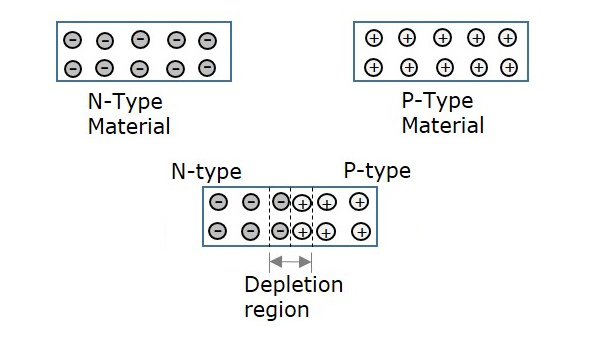Hence, the current formed due to the diffusion of these electrons and holes, without the application of any kind of external energy, can be termed as Diffusion Current.

### Drift Current

The current formed due to the drift (movement) of charged particles (electrons or holes) due to the applied electric field, is called as Drift Current. The following figure explains the drift current, whether how the applied electric field, makes the difference.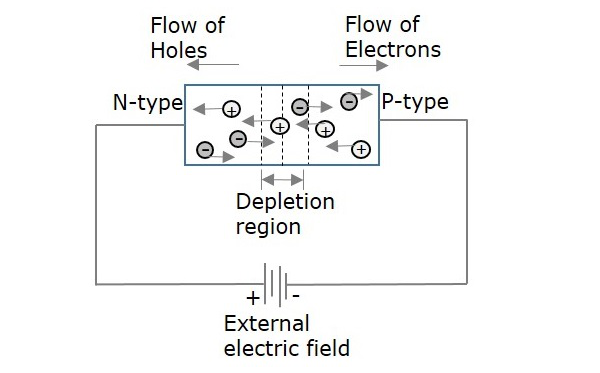The amount of current flow depends upon the charge applied. The width of depletion region also gets affected, by this drift current. To make a component function in an active circuit, this drift current plays an important role.